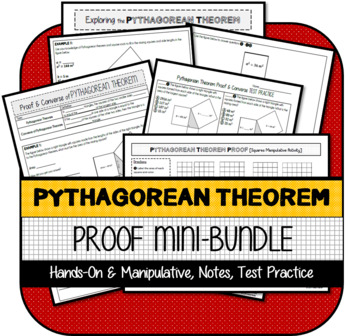# Pythagorean Theorem Proof Mini-BundleSubject
Resource Type
File Type

Zip

(1 MB|9+)
Product Rating
4.0
(1 Rating)
Standards
3 Products in this Bundle
3 products
1. This resource was developed to meet the requirements of the 8th Grade Geometry Standard below:CCSS.MATH.CONTENT.8.G.B.6Explain a proof of the Pythagorean Theorem and its converse.It contains:1) Pythagorean theorem Proof figure with squares to scale of “Starburst” candies 2) Optional cut-out manipula
2. This is a simple activity designed to reinforce the Pythagorean theorem and emphasize that it is the sum of the SQUARES of the side lengths, not the side lengths themselves, that total the SQUARE of the hypotenuse. Best used after an introduction activity. Most effectively completed when students a
3. This resource was developed to meet the requirements of the 8th Grade Geometry Standard below:CCSS.MATH.CONTENT.8.G.B.6 Explain a proof of the Pythagorean Theorem and its converse.It contains the following resources:1) Pythagorean Theorem Proof & Converse: 4 Example Problems 2) Pythagorean Theo
Also included in:
1. This product was created to introduce, support, and assess students with the following 8th Grade Geometry Standards:CCSS.MATH.CONTENT.8.G.B.6Explain a proof of the Pythagorean Theorem and its converse.CCSS.MATH.CONTENT.8.G.B.7Apply the Pythagorean Theorem to determine unknown side lengths in right t
\$17.00
\$12.00
Save \$5.00
2. This product includes:1) Notes2) Practice3) Games4) Activities5) Test Practice6) Quizzes7) Assessments8) Google Apps Optionsfor ALL 8th Grade Geometry for Common Core Standards. LICENSING TERMS: This purchase includes a license for one teacher only for personal use in their classroom. Licenses are n
\$69.50
\$50.00
Save \$19.50
• Bundle Description
• StandardsNEW

This resource was developed to meet the requirements of the 8th Grade Geometry Standard below:

CCSS.MATH.CONTENT.8.G.B.6
Explain a proof of the Pythagorean Theorem and its converse.

This mini-bundle of activities is MOST EFFECTIVE when used in the following order:

1) Pythagorean Theorem Proof Introduction: Hands-On Exploration

2) Pythagorean Theorem Proof: Squares Manipulative Activity

3) Pythagorean Theorem Proof & Converse NOTES & TEST PRACTICE

Note: It does NOT contain a reference to the Pythagorean theorem formula, however this serves as a building block between the concrete and abstract.

LICENSING TERMS: This purchase includes a license for one teacher only for personal use in their classroom. Licenses are non-transferable, meaning they can not be passed from one teacher to another. No part of this resource is to be shared with colleagues or used by an entire grade level, school, or district without purchasing the proper number of licenses.

COPYRIGHT TERMS: This resource may not be uploaded to the internet in any form, including classroom/personal websites or network drives, unless the site is password protected and can only be accessed by students.

Explain a proof of the Pythagorean Theorem and its converse.
Total Pages
9+
Included
Teaching Duration
3 hours
Report this Resource to TpT
Reported resources will be reviewed by our team. Report this resource to let us know if this resource violates TpT’s content guidelines.